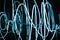# ACTIVATION FUNCTIONSThe art of non-linearity

This article is a brief discussion of the following sections

• Activation function
• Various activation functions
• Need for an activation function

# Activation function

Activation functions are the equations that determine the output of a neural network. The main purpose of an activation function is to introduce non-linearity to the neural network.

• An activation function converts that linear input of a neuron to a non-linear output.
• It helps in normalizing the output of each neuron between the range of -1 to1

# Different Activation functions

• Sigmoid Function
• Softmax Function
• Hyperbolic Tangent Function (Tanh)
• Rectified Linear Unit Function (ReLU)
• Exponential Linear Unit Function (ELU)
• Leaky Rectified Linear Unit Function…

# Bayes’ theorem

Probability is nothing but how likely an event is to occur

Event → It is an outcome of an experiment or an outcome that we experience

For example, consider you are rolling a die

Experiment → rolling a 6 sided die

Event → The outcome after rolling the die is 4

`Possible outcomes after rolling the die = [1,2,3,4,5,6]Total number of outcomes = 6`

How likely that the outcome is 4 is the probability of the event = 4

`P(Event = 4) = (no_of_outcomes = 4)/(total_no_outcomes) = 1/6`

Similarly considering the above experiment

`P(Event = 1) = 1/6P(Event…`

# Sorting in python

## What’s sorting?

Sorting is nothing but arranging the items in a particular sequence. In other words, sorting is the ordering of elements based on our preference. Preference can be ascending or descending depending on our requirements.

In this article, we are going to discuss two sorting algorithms and their differences.

1. Insertion sort
2. Merge sort

# Insertion sort

Consider a list of elements

`x = [73, 79, 56,  4, 48, 35]`

Implementation strategy for ascending order

Step 1:

`key_element = x[key]x[:key] is the list that contains all the elements before the key elementx[:key] is sorted`

Step 2:

`if some of the elements in x[:key]…`

# N-Gram Language Models

In this article, we are going to discuss language modeling, generate the text using N-gram Language models, and estimate the probability of a sentence using the language models. First of all, what is language modeling?

Language Modeling is nothing but a process of predicting what word comes next.

A language model learns the probability of word occurrence based on examples of text or the training data

Consider a sequence of words

`x1, x2, x3, x4,...,xn`

Assume your Vocabulary set is V and m words in it

`V = {w1, w2, w3,...,wm}`

Compute the probability distribution of the next word x(n+1)…

# Markov Chains

In this article, we are going to discuss Markov chains, their properties, and their implementation in python. First, let’s try to get an intuition of what exactly is a Markov property?

A Markov property is an assumption that future states depend only on the present state, not on the states that occurred in the past.

A Markov chain is a stochastic process that satisfies the Markov Property

Consider a system of 5 states

`S = {1, 2, 3, 4, 5}`

The state of a Markov Chain at time t is the value of Xt.

For example, if Xt = 2…

# Covariance and Correlation

In this article, we are going to discuss the concepts of Covariance and Correlation in terms of Statistics and implement them in python.

Covariance is a metric that gives us the relationship between two random variables. This metric evaluates how much the variables change together. It is nothing but a measure of the variance between two variables.

Before diving into covariance we need to understand about mean and variance

Mean or average or expected value is the central value of a set of numbers i.e sum of the values divided by the number of values

Consider a random variable X…

# The Concept of Error Analysis

If you are starting to work on a Machine Learning Problem or building a machine learning application, starting with a simple algorithm that you can implement quickly and test it with your validation dataset is always considered the best practice

Plot Learning curves, error analysis which is manually looking at the errors(the examples in the validation dataset that the simple algorithm doesn’t work properly) to generate more insights.

With the help of error analysis, you can try different ideas and cross-check whether they are improving your application or not.

If your dataset consists of skewed classes it’s much harder to…

# Entropy, Loss Functions and the Mathematical Intuition behind them

A Loss Function is an essential step in any Deep Learning Problem. First of all, what is a loss function?

A Loss function is just an evaluation method that gives information about how well your model is working. If the predictions using the model are totally different from the true values then the loss function outputs a larger number. As you make changes to improve your model, loss function will tell you whether the model is actually showing any improvements on the given dataset or not.

A loss function gives an idea about how good our classifier is and it…

# Features of Pandas and their functionality

Every problem related to Data Science starts with Exploratory Data Analysis(EDA). So what’s EDA?

EDA or Exploratory Data Analysis is an approach for Data Analysis that employs various techniques to get as many insights as possible form the existing data. Some of the Insights are detecting important variables, detecting outliers, etc

My journey with EDA has been possible mainly with the help of two libraries (pandas and NumPy) and the features associated with them. We will explore some of the features and the functionality of Pandas(Since Pandas depends upon and interoperates with NumPy)

## Creating a series with some random values

`np.random.seed(1)X = np.random.randint(low=1, …`ML Engineer